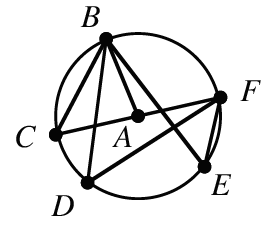### Home > GC > Chapter 10 > Lesson 10.1.2 > Problem10-17

10-17.

In $⊙A$ at right, $\overline{CF}$, is a diameter and $m∠C = 64°$. Find:1. $m \angle D$

How is $∠D$ related to $∠C$?

$∠D$ and $∠C$ are both inscribed angles with the same intercepted arc. So, $∠D ≅ ∠C = 64^{\large\circ}$.

1. $m\overarc{ B F }$

Use the same method you used for part (a).

The measure of the intercepted arc is twice the measure of the inscribed angle. So, the measure is $128^{\large\circ}$.

1. $m∠E$

$64^{\large\circ}$

1. $m\overarc{ C B F }$

1. $m∠BAF$

1. $m∠BAC$